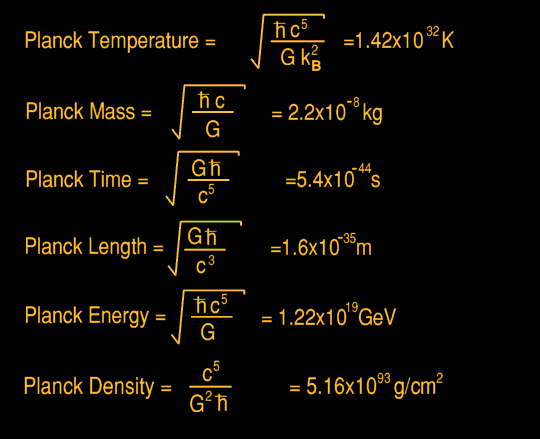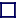### PLANCK BLACK HOLES

PLANCK MASS LENGTH AND TIME
Using just three universal constants, the speed of light c, Planck's constant h-bar, and Newtons gravitational constant G, three other fundamental constants can be mathematically constructed having the units of mass, length and time: the Planck mass, Planck length and Planck time, taking the values of 2.17×10-5 grams, 1.62×10-35 metres, and 5.39×10-44 seconds respectively. The last two represent the absolute minimum values of length and time. Space and time are quantised. The first is not; masses less than the Planck mass do indeed exist. The Planck time is that duration of time which a photon, travelling at lightspeed, takes to travel the Planck length. According to Heisenbergs' Uncertainty Principle, uncertainties in length are inversely proportional to uncertainties in energy. So, in order to explore such short distances, an energy of 1.22×1016 TeV (the 'Planck' energy) would be required, corresponding to a temperature of 1.42×1032 Kelvin (the 'Planck' temperature). These energies are so extreme as to be 1020 times greater than those which our present most powerful particle accelerators can reach and represents the energy where space and time become unstable and worm-holes start to appear.

There is also a corresponding Planck Density, being 5.16×1093gm/cm3 which cannot be exceeded, and the Planck Force, c4G, which cannot be surpassed. The Planck force is equal to 1.21027×1044Newtons (approximately 1040tons) and if strings exist would be the tension in the strings of String Theory. This also implies a Planck acceleration, which is 4.9223 ×10102m/s2. The Planck Frequency is the reciprocal of Planck Time, and equal to 1.885487×1043radians/second. A Planck voltage can be derived, being 1.04295×1027volts, as can a Planck current of 3.4789×1025Amps, and therefore also a Planck power of 3.62831×1052watts. Dividing the Planck Voltage by the Planck current gives a Planck impedance, or resistance, of just 29.792458 ohms, which certainly can be exceeded!

Although the values for the speed of light, c and h-bar are known with some precision, the value of G, the Universal constant of Gravitation, is much less well known. Measurement of this is particularly difficult, so this limits the known accuracies of the Planck units. The value of G is approximately 66.7259×10-12 m3kg-1s-2.

The minimum size of a Black Hole is one which has such a mass that it entirely evaporates by Hawking thermal radiation in the Planck time. Such a Black Hole has a Schwarzschild radius equal to the Planck length and a mass equal to the Planck mass, and this radiates Hawking thermal radiation at a characteristic Black Body temperature equal to the Planck temperature yielding an energy equal to the Planck energy. At such fundamental Planck distances, space is thought to exist as a seething sea of virtual Planck Black Holes, giving a dis-continuous foamy structure to space itself. A Planck Black Hole seems to have quantum properties suggestive of a fundamental particle, albeit, of the utmost in-stability. However, so far, a quantum theory of gravity has eluded physicists.

 WHY TIME & SPACE ARE QUANTISED To measure distance to such shorter lengths (shorter than the Planck Length) would require an energy above that of the Planck Energy to be concentrated in a Length shorter than the Planck Length, which is just not possible. It cannot be done. Time jumps from one Planck interval to the next at a frequency of about 1043 Hz. This also means that that is the maximum possible frequency, the Planck Frequency. Any photon of that frequency (energy) would have a mass equal to the Planck Mass and thus turn itself into a Planck Black Hole for a length of time equivalent to the Planck Time, before exploding again turning itself into a photon of Planck Mass which turns itself into a Planck Black hole, which explodes and... This photon travels in discrete distance intervals, and discrete time intervals. It does not travel linearly. It is following the Planck Time interval in steps of the Planck length. It doesn't even know whether its a photon or a black hole. At such Planck energies time and space are seething.

The Author rather likes the possibility that a Black Hole itself is a thin hollow sphere with nothing inside, not even space. The thin shell is the thinnest possible thickness, the Plank Length, and it is at a temperature of the Plank Temperature. Only the size of the sphere changes in relation to its mass, all of which is concentrated into this thin shell, there being no singularity at the centre.

That is just one possibility, one the Author favours. Thus a large Black Hole consists of trillions of minuscule Plank Black Holes, all arranged as the shell of a hollow sphere, and all on the Event Horizon. This arrangement has several properties that ordinary Black Holes lack; nothing can go beyond the Event Horizon, and ordinary matter is obliterated the moment it strikes the tremendously hot thin outer shell of the Event Horizon. The Event Horizon is at the highest temperature anything can ever be. This is the so-called 'firewall' of a Black Hole through which nothing can travel.

If there is no inside part of a Black Hole (nor of a Planck Black Hole), then perhaps the shell at the Event Horizon, as well as being the thinnest possible thickness, has only one side - it's topography is that of a Klein bottle, where the surface of a sphere, like a Mobius strip in two dimensions, folds back on itself after a twist, resulting in a 3-dimensional vessel with only one surface: an outer surface only. There is no inner surface.

This topography has parallels with the topography of fundamental particles in Rogers' Twisted Triprism Ring Theory, where twists in three dimensions bestow electrons with three sides and quarks with only one side.